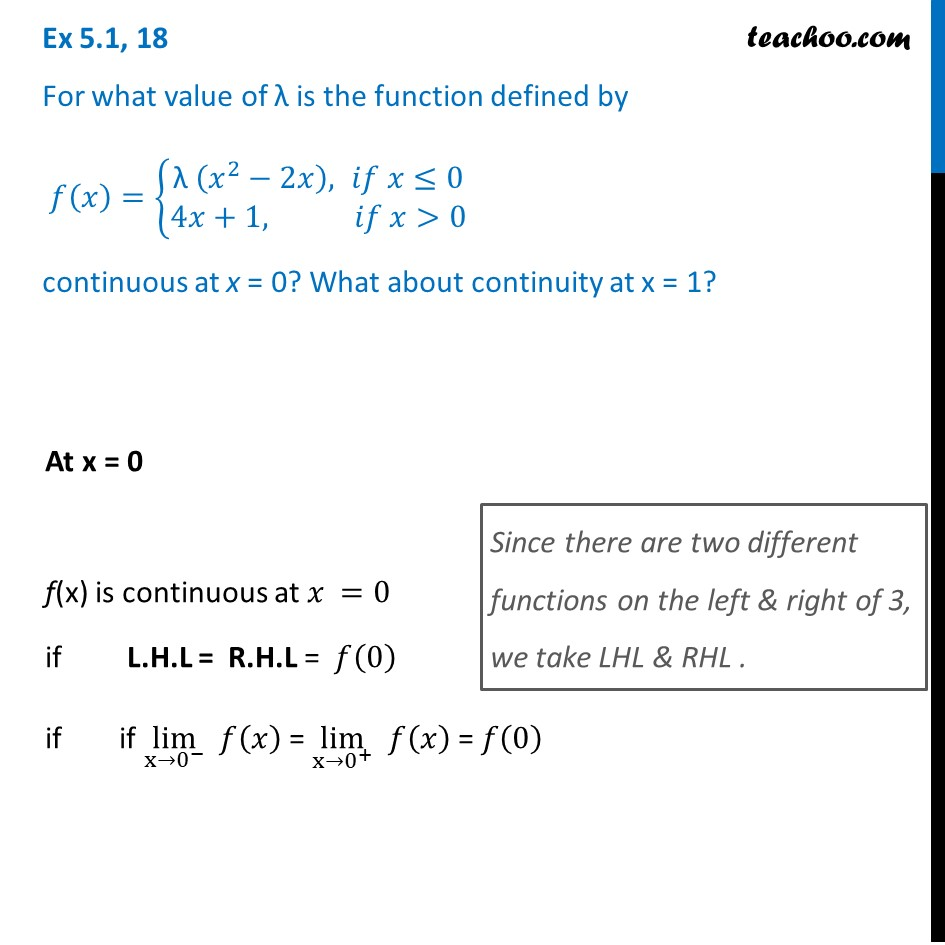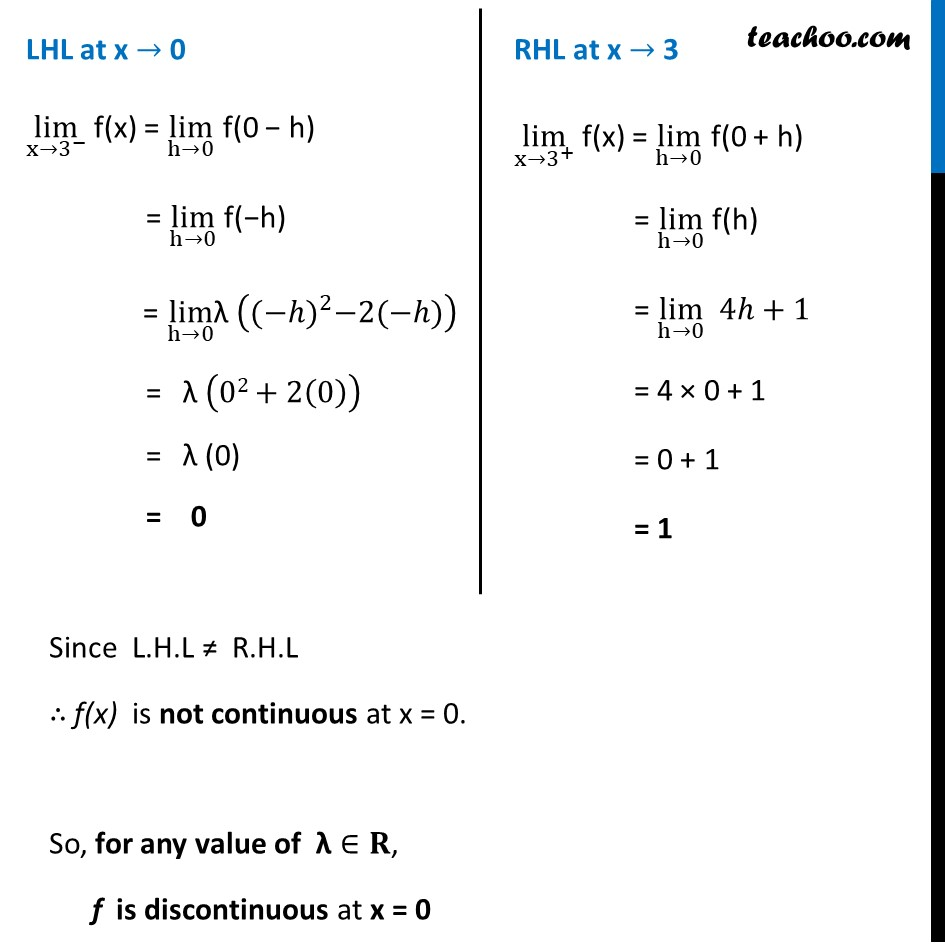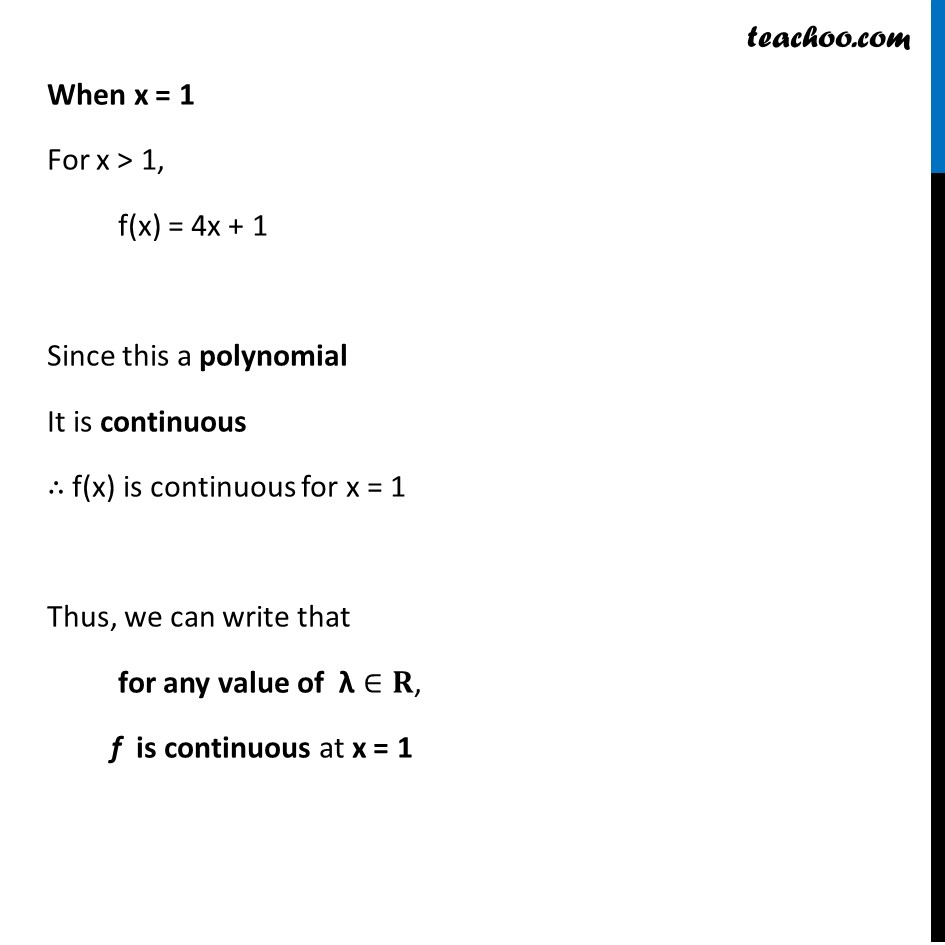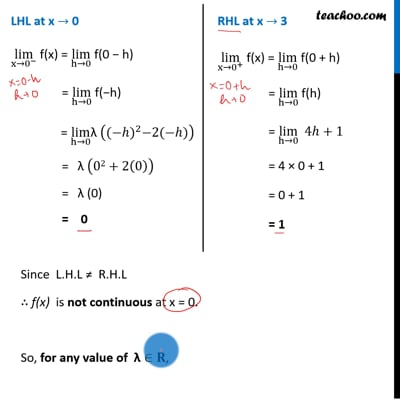Ex 5.1

Chapter 5 Class 12 Continuity and Differentiability
Serial order wiseThis video is only available for Teachoo black users

Solve all your doubts with Teachoo Black (new monthly pack available now!)

### Transcript

Ex 5.1, 18 For what value of λ is the function defined by 𝑓(𝑥)={█("λ" (𝑥^2−2𝑥), 𝑖𝑓 𝑥≤0@&4𝑥+1, 𝑖𝑓 𝑥>0)┤ continuous at x = 0? What about continuity at x = 1? At x = 0 f(x) is continuous at 𝑥 =0 if L.H.L = R.H.L = 𝑓(0) if if lim┬(x→0^− ) 𝑓(𝑥) = lim┬(x→0^+ ) 𝑓(𝑥) = 𝑓(0) Since there are two different functions on the left & right of 3, we take LHL & RHL . LHL at x → 0 lim┬(x→3^− ) f(x) = lim┬(h→0) f(0 − h) = lim┬(h→0) f(−h) = lim┬(h→0) "λ" (〖(−ℎ)〗^2−2(−ℎ)) = "λ" (02+2(0)) = "λ (0)" = 0 RHL at x → 3 lim┬(x→3^+ ) f(x) = lim┬(h→0) f(0 + h) = lim┬(h→0) f(h) = lim┬(h→0) 4ℎ+1 = 4 × 0 + 1 = 0 + 1 = 1 Since L.H.L ≠ R.H.L ∴ f(x) is not continuous at x = 0. So, for any value of "λ"∈𝐑, f is discontinuous at x = 0 When x = 1 For x > 1, f(x) = 4x + 1 Since this a polynomial It is continuous ∴ f(x) is continuous for x = 1 Thus, we can write that for any value of "λ"∈𝐑, f is continuous at x = 1